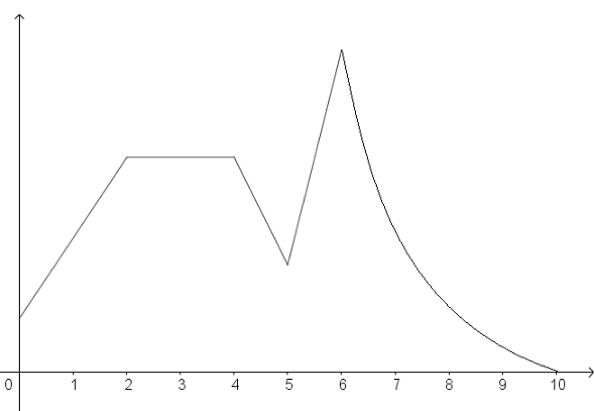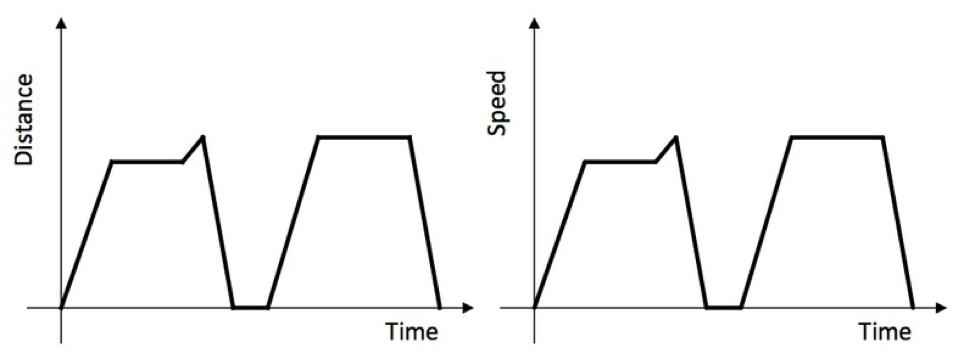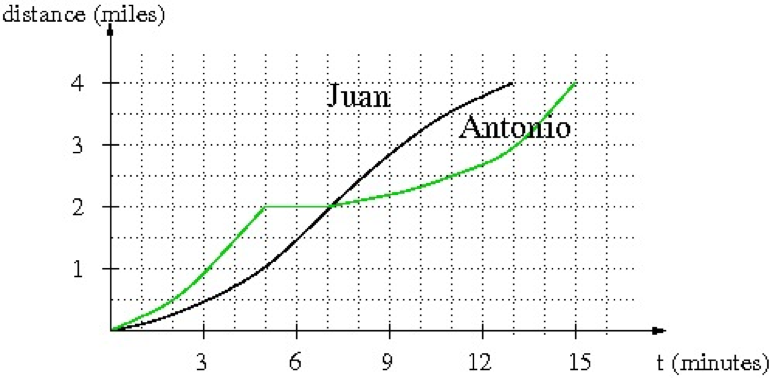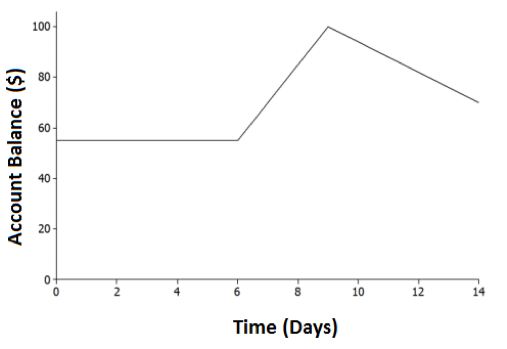# Functions

## Objective

Describe functions by analyzing graphs. Identify intervals of increasing, decreasing, linear, or nonlinear activity.

## Common Core Standards

### Core Standards

?

• 8.F.B.5 — Describe qualitatively the functional relationship between two quantities by analyzing a graph (e.g., where the function is increasing or decreasing, linear or nonlinear). Sketch a graph that exhibits the qualitative features of a function that has been described verbally.

## Criteria for Success

?

1. Identify and describe where a graph may be increasing or decreasing.
2. Identify and describe where a graph may be linear or nonlinear.
3. Interpret a graph in context of the situation, paying close attention to the variables involved.
4. Describe stories that match graphical representations of functions.

## Tips for Teachers

?

Lessons 11 and 12 focus on graphical representations of functions and understanding them qualitatively. In Lesson 11, students analyze the structural appearance of graphs to understand and describe how the behavior of the function changes (MP.7). In Lesson 12, students sketch graphs when given descriptions.

#### Fishtank Plus

• Problem Set
• Student Handout Editor
• Vocabulary Package

## Anchor Problems

?

### Problem 1

Use the graph of the function below to answer the questions that follow.1. Name an interval of $x$ where the function is linear.
2. Name an interval of $x$ where the function is increasing.
3. Name an interval of $x$ where the function is decreasing linearly.
4. In what interval of $x$ is the function increasing at its greatest rate?
5. Explain what is happening in the interval where $x$ is between 2 and 4.

### Problem 2

Below are two graphs that look the same. Note that the first graph shows the distance of a car from home as a function of time, and the second graph shows the speed of a different car as a function of time.

Describe what someone who observes each car’s movement would see in each case.#### References

Illustrative Mathematics Distance

Distance, accessed on Oct. 27, 2017, 10:54 a.m., is licensed by Illustrative Mathematics under either the CC BY 4.0 or CC BY-NC-SA 4.0. For further information, contact Illustrative Mathematics.

### Problem 3

Antonio and Juan are in a 4-mile bike race. The graph below shows the distance of each racer (in miles) as a function of time (in minutes).1. Who wins the race? How do you know?
2. Imagine you were watching the race and had to announce it over the radio; write a little story describing the race.

#### References

Illustrative Mathematics Bike Race

Bike Race, accessed on Oct. 27, 2017, 10:55 a.m., is licensed by Illustrative Mathematics under either the CC BY 4.0 or CC BY-NC-SA 4.0. For further information, contact Illustrative Mathematics.

## Problem Set

?The following resources include problems and activities aligned to the objective of the lesson that can be used to create your own problem set.

?

Use the graph below to answer the questions that follow.1. Which statements are true? Select all that apply.
1. The function is linear and increasing between days 0 and 9.
2. The function is linear and decreasing between days 9 and 14.
3. The function is nonlinear between days 0 and 6.
4. The function is neither increasing nor decreasing between days 0 and 6.
5. The function decreases for more days than it increases.

1. Which description is best represented by the graph?
1. Adan added the same amount of money to his savings account for 6 days. Then, for the next 3 days, he increased how much he added to the account each day. Lastly, he decreased the amount of money he added to his account each day.
2. Adan started with about \$55 dollars in his savings account. He didn’t add anything to it for 6 days. Then he got some birthday money and added money to his savings account over 3 days, after which he started to take money out of his account to make purchases.

#### References

EngageNY Mathematics Grade 8 Mathematics > Module 6 > Topic A > Lesson 4Exercise 4

Grade 8 Mathematics > Module 6 > Topic A > Lesson 4 of the New York State Common Core Mathematics Curriculum from EngageNY and Great Minds. © 2015 Great Minds. Licensed by EngageNY of the New York State Education Department under the CC BY-NC-SA 3.0 US license. Accessed Dec. 2, 2016, 5:15 p.m..

Modified by The Match Foundation, Inc.

?